# Selina Solutions Concise Maths Class 10 Chapter 19 Constructions (Circles)

In this chapter, students will learn the methods of construction of tangents to a given circle, construction of circumscribed and inscribed circles of a triangle and constructing a circumscribed and inscribed circle on a regular hexagon. Understanding these constructions are very essential as at least one question is expected from this chapter in the ICSE. So, students having any difficulties in understanding any chapter of Concise Mathematics Selina for Class 10 can refer to the Selina Solutions for Class 10 Mathematics. Further, students can access the Selina Solutions for Class 10 Mathematics Chapter 19 Constructions (Circles) free PDF to enhance their preparations for their examinations.

## Selina Solutions Concise Maths Class 10 Chapter 19 Constructions (Circles) Download PDF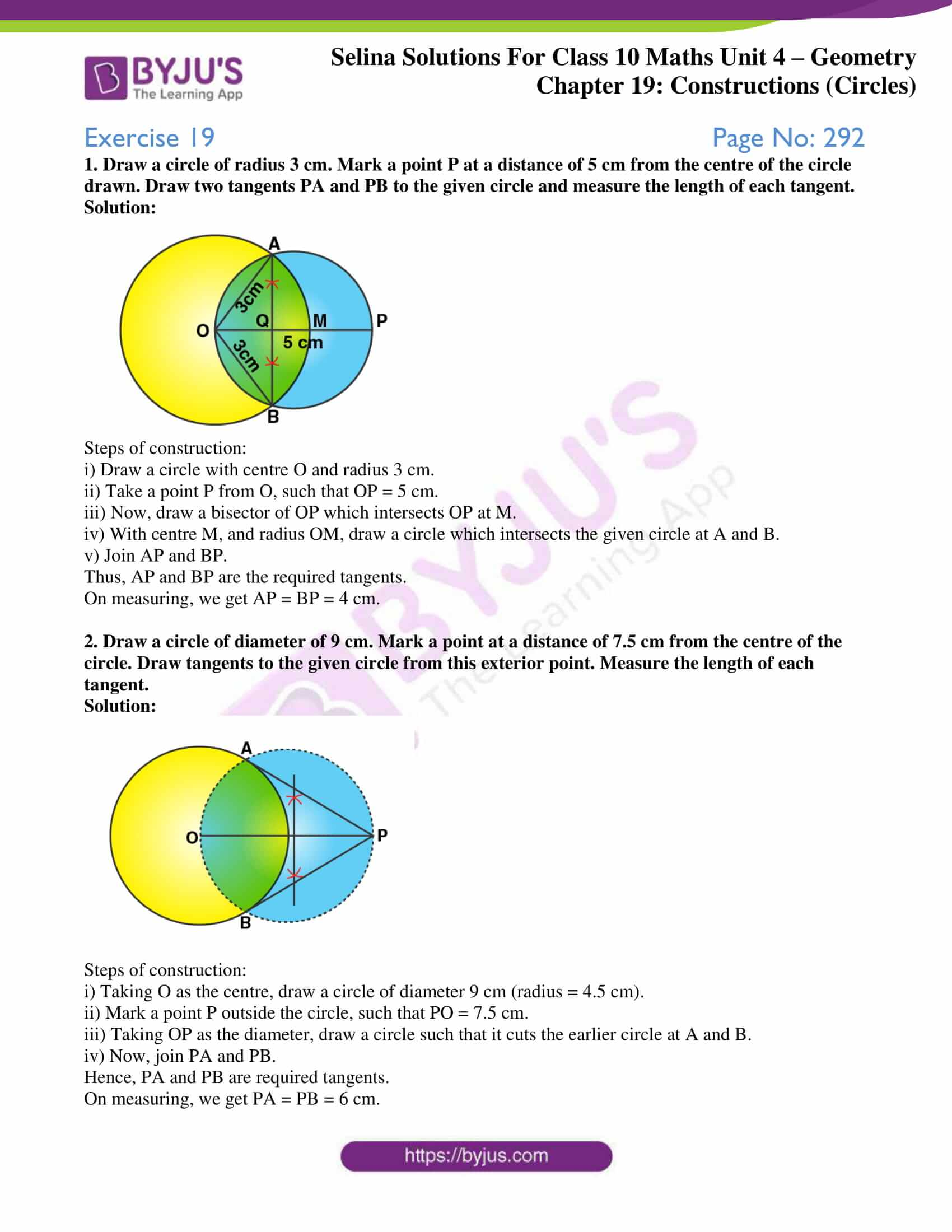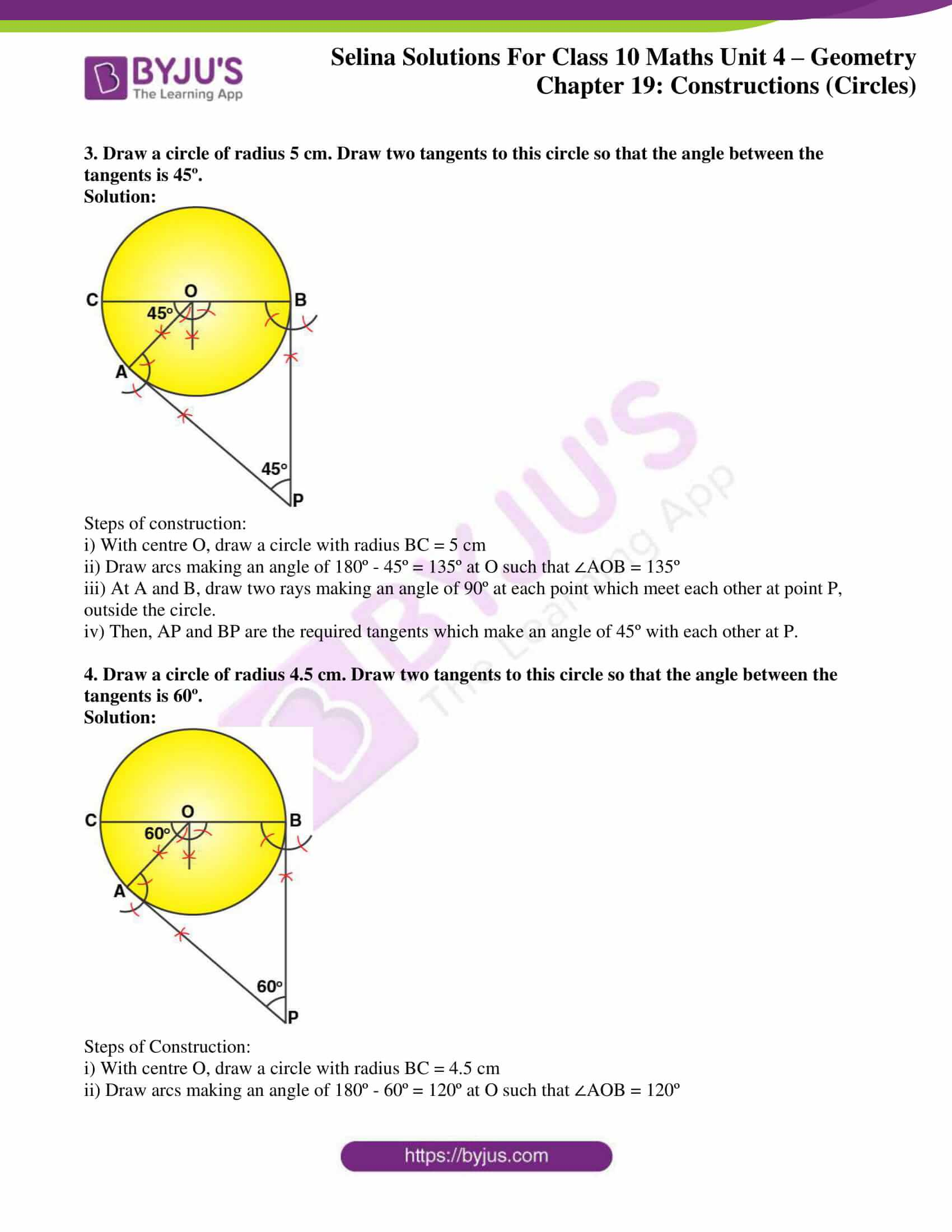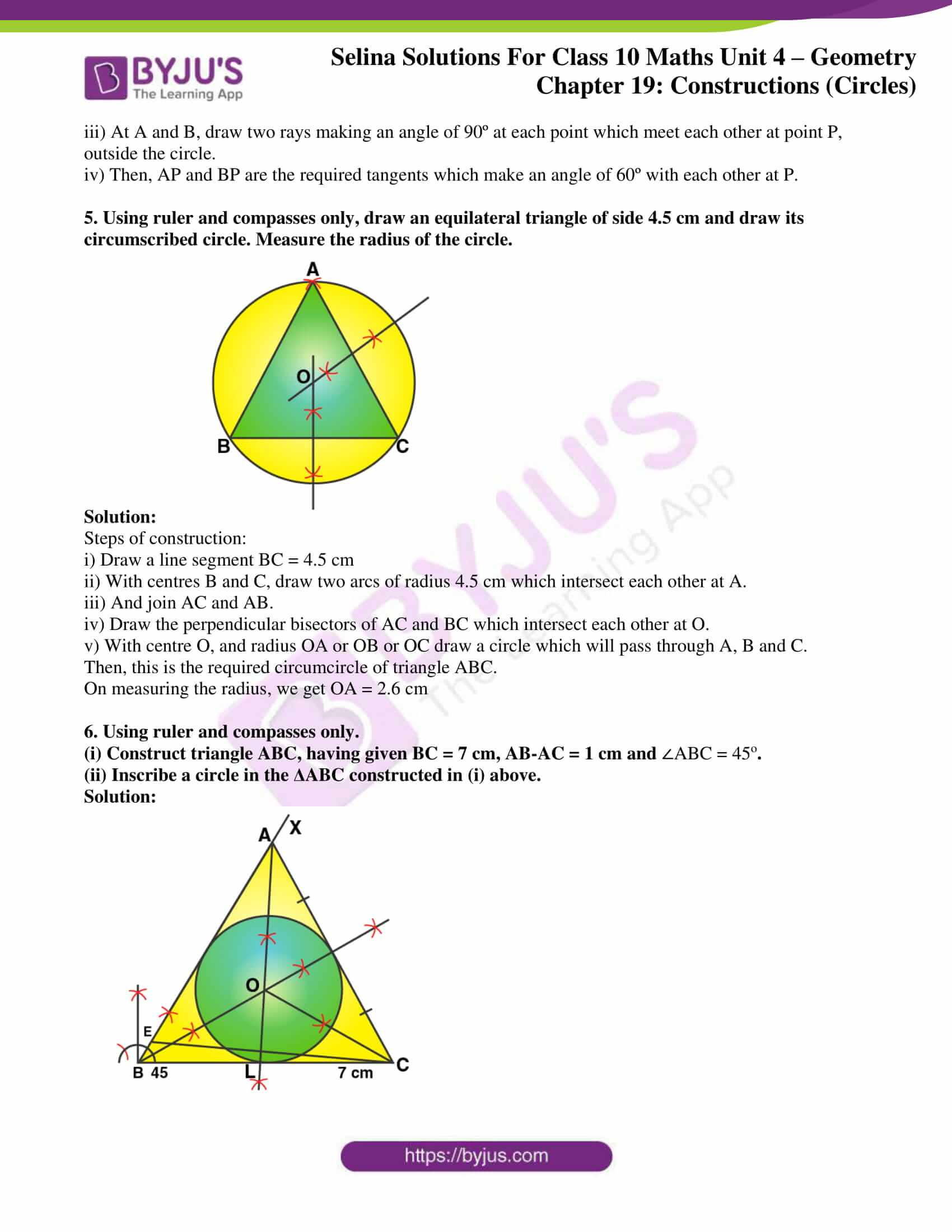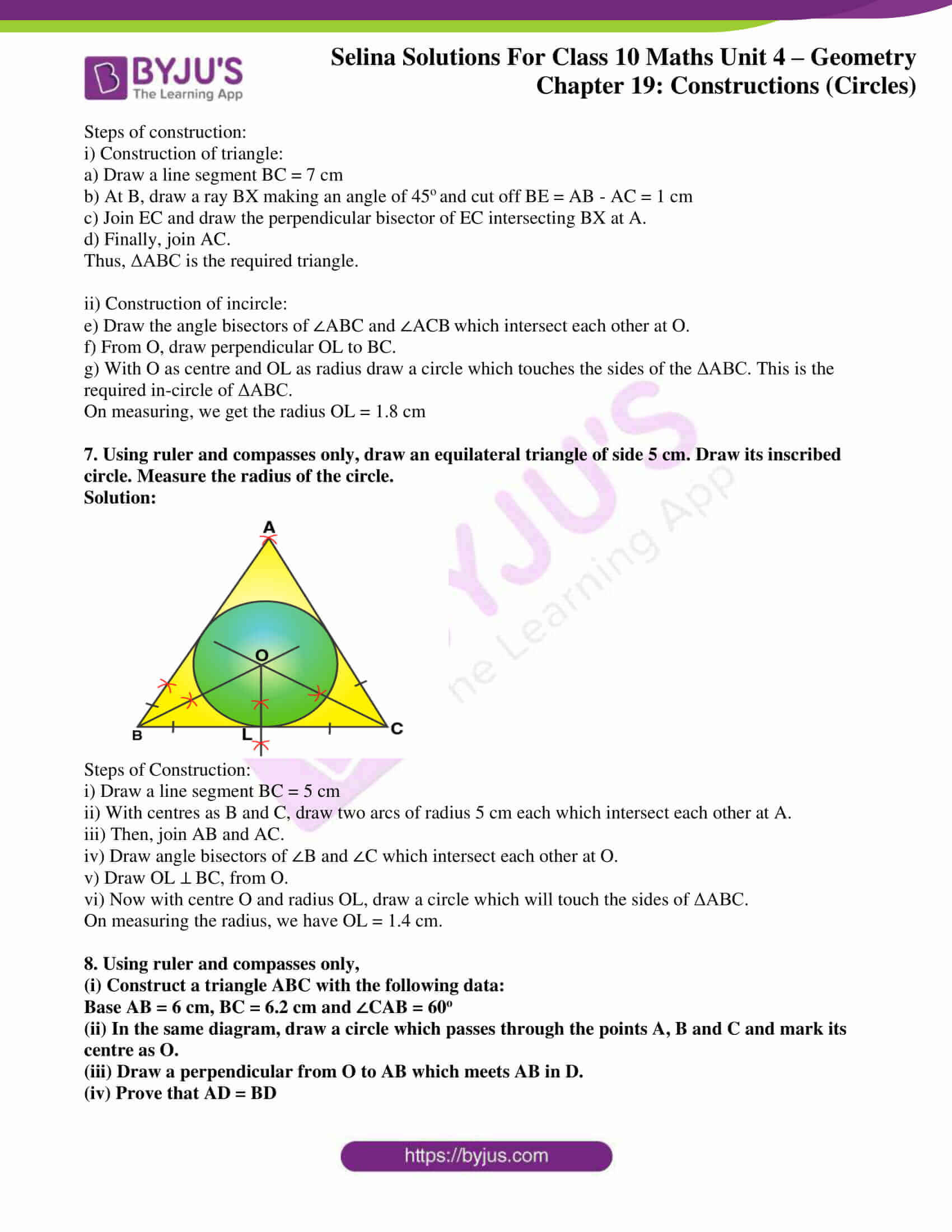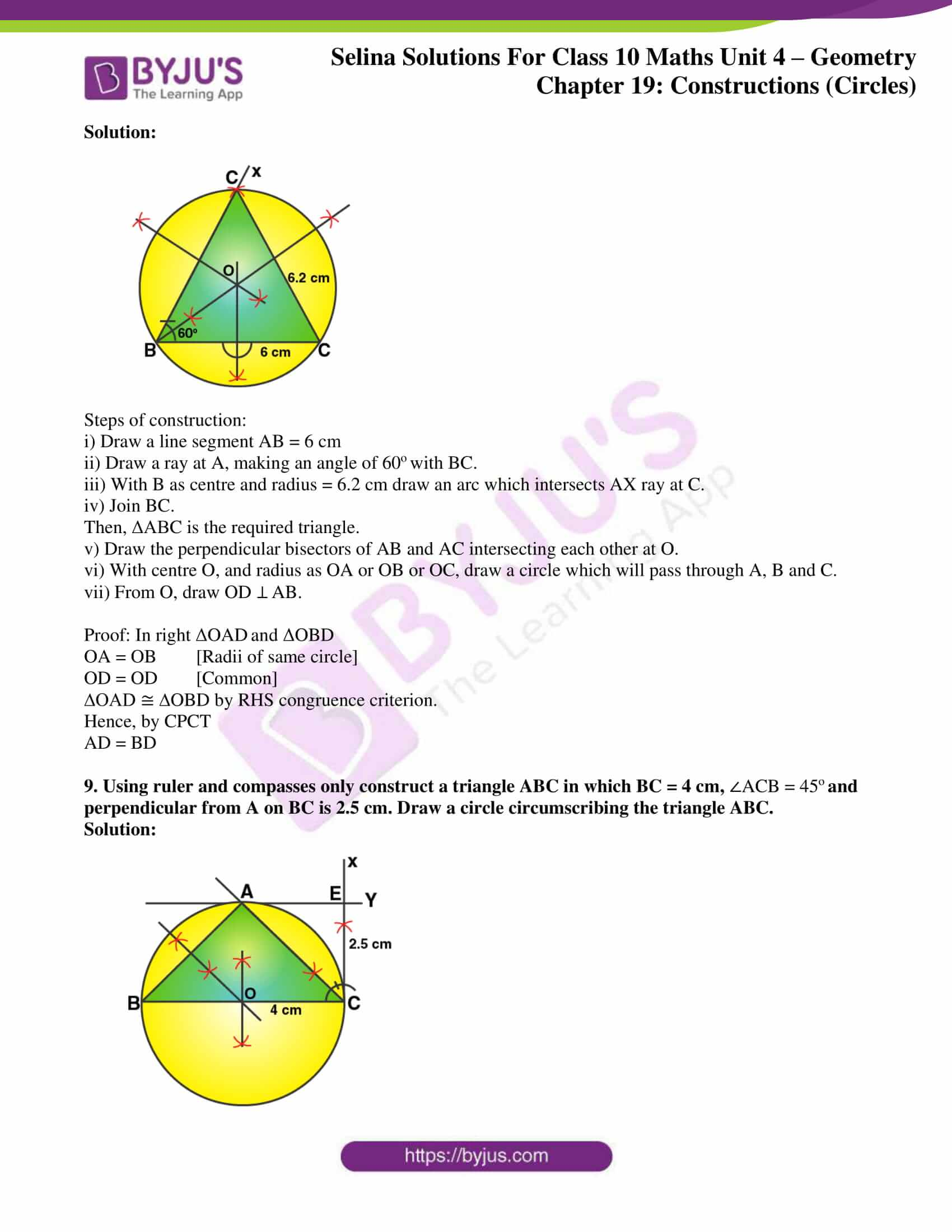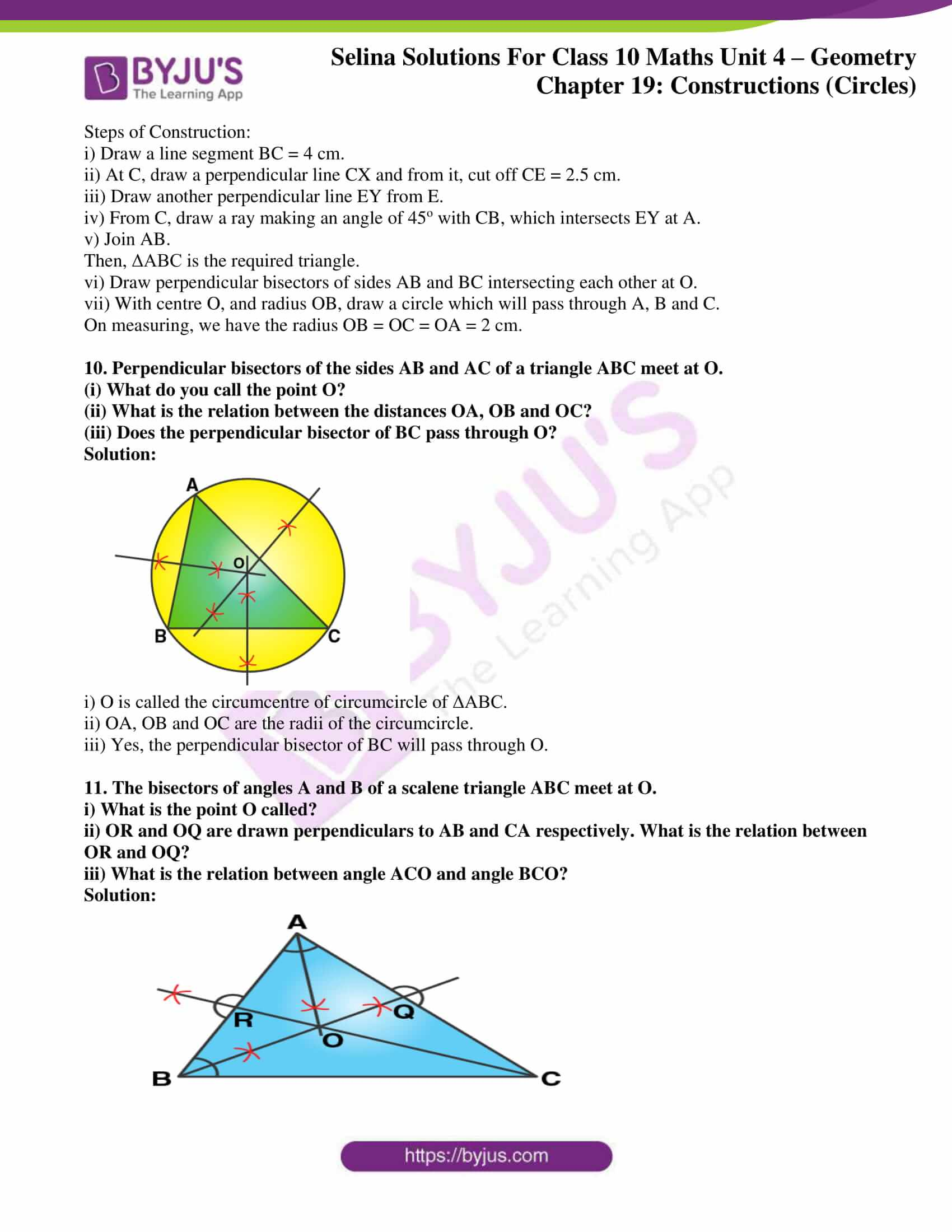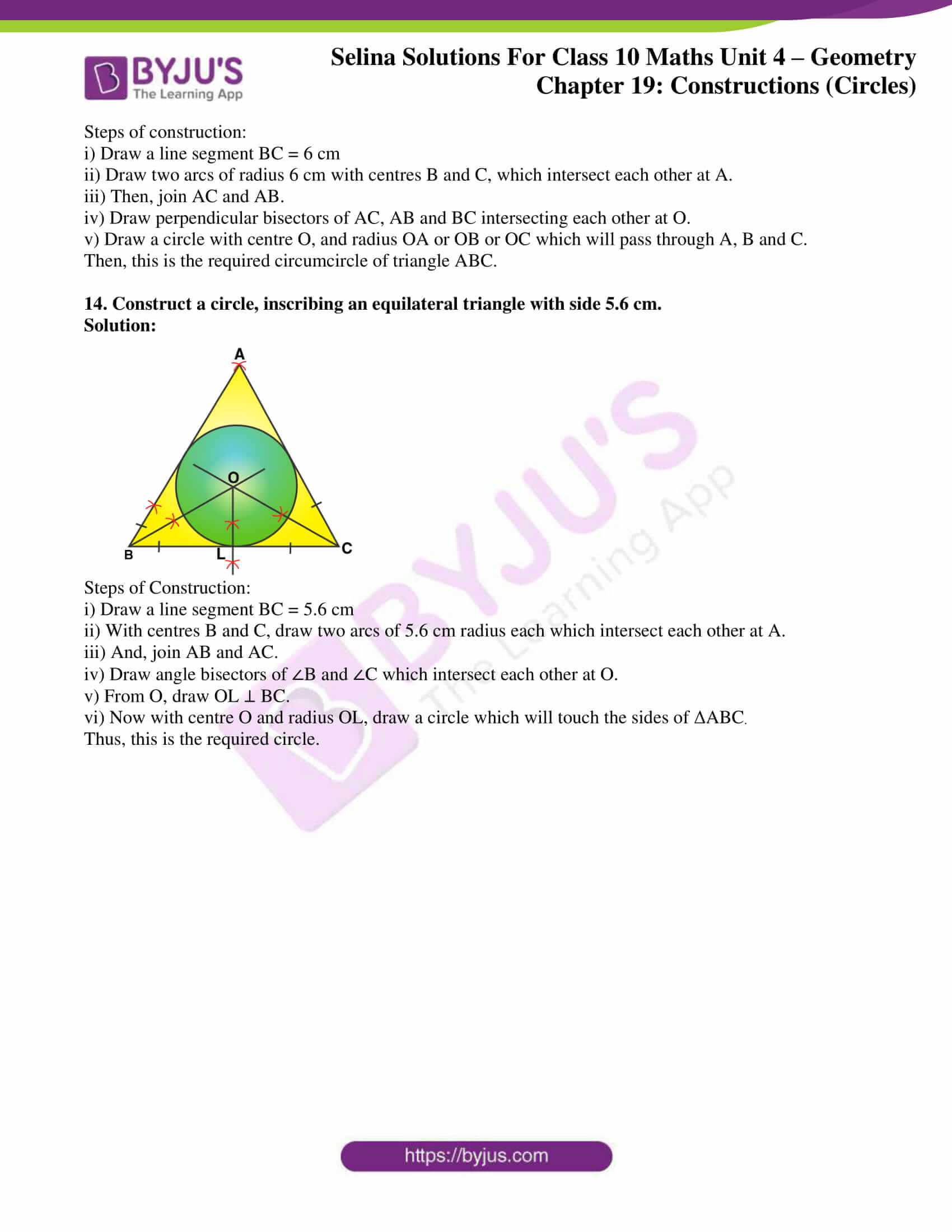## Access Selina Solutions Concise Maths Class 10 Chapter 19 Constructions (Circles)

Exercise 19 Page No: 292

1. Draw a circle of radius 3 cm. Mark a point P at a distance of 5 cm from the centre of the circle drawn. Draw two tangents PA and PB to the given circle and measure the length of each tangent.

Solution: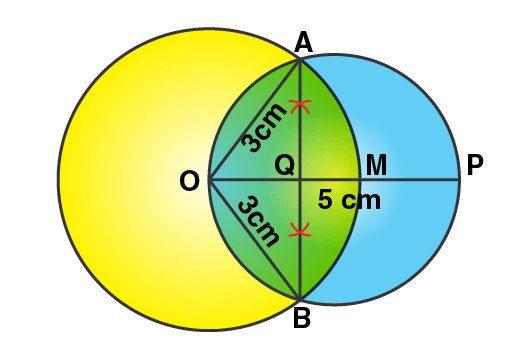Steps of construction:

i) Draw a circle with centre O and radius 3 cm.

ii) Take a point P from O, such that OP = 5 cm.

iii) Now, draw a bisector of OP which intersects OP at M.

iv) With centre M, and radius OM, draw a circle which intersects the given circle at A and B.

v) Join AP and BP.

Thus, AP and BP are the required tangents.

On measuring, we get AP = BP = 4 cm.

2. Draw a circle of diameter of 9 cm. Mark a point at a distance of 7.5 cm from the centre of the circle. Draw tangents to the given circle from this exterior point. Measure the length of each tangent.

Solution: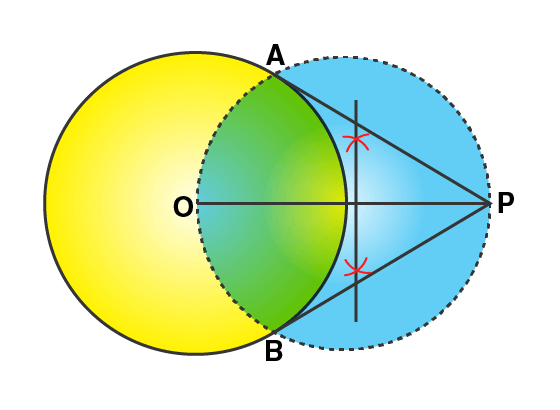Steps of construction:

i) Taking O as the centre, draw a circle of diameter 9 cm (radius = 4.5 cm).

ii) Mark a point P outside the circle, such that PO = 7.5 cm.

iii) Taking OP as the diameter, draw a circle such that it cuts the earlier circle at A and B.

iv) Now, join PA and PB.

Hence, PA and PB are required tangents.

On measuring, we get PA = PB = 6 cm.

3. Draw a circle of radius 5 cm. Draw two tangents to this circle so that the angle between the tangents is 45º.

Solution: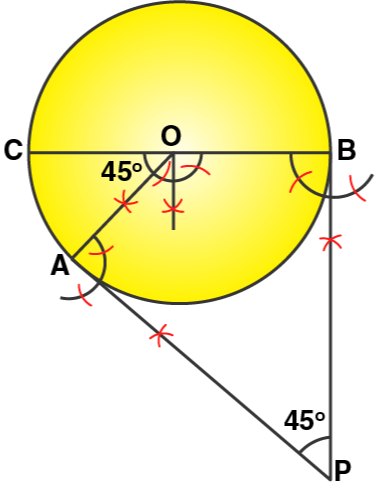Steps of construction:

i) With centre O, draw a circle with radius BC = 5 cm

ii) Draw arcs making an angle of 180º – 45º = 135º at O such that ∠AOB = 135º

iii) At A and B, draw two rays making an angle of 90º at each point which meet each other at point P, outside the circle.

iv) Then, AP and BP are the required tangents which make an angle of 45º with each other at P.

4. Draw a circle of radius 4.5 cm. Draw two tangents to this circle so that the angle between the tangents is 60º.

Solution: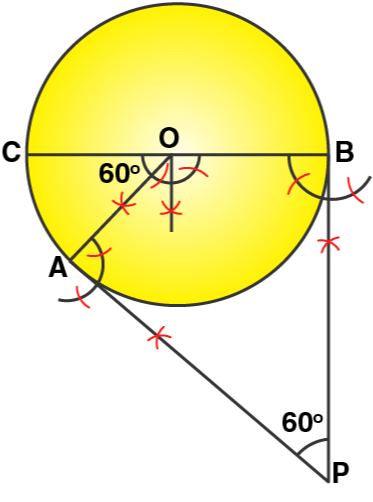Steps of Construction:

i) With centre O, draw a circle with radius BC = 4.5 cm

ii) Draw arcs making an angle of 180º – 60º = 120º at O such that ∠AOB = 120º

iii) At A and B, draw two rays making an angle of 90º at each point which meet each other at point P, outside the circle.

iv) Then, AP and BP are the required tangents which make an angle of 60º with each other at P.

5. Using ruler and compasses only, draw an equilateral triangle of side 4.5 cm and draw its circumscribed circle. Measure the radius of the circle.

Solution: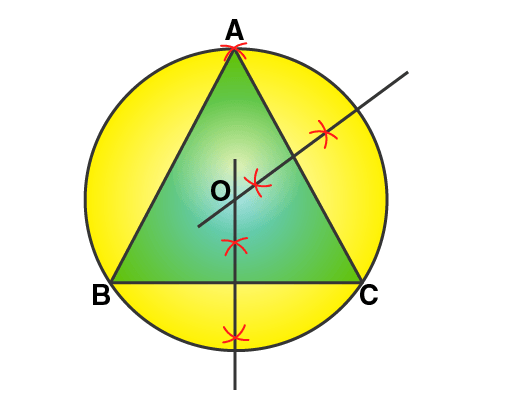Steps of construction:

i) Draw a line segment BC = 4.5 cm

ii) With centres B and C, draw two arcs of radius 4.5 cm which intersect each other at A.

iii) And join AC and AB.

iv) Draw the perpendicular bisectors of AC and BC which intersect each other at O.

v) With centre O, and radius OA or OB or OC draw a circle which will pass through A, B and C.

Then, this is the required circumcircle of triangle ABC.

On measuring the radius, we get OA = 2.6 cm

6. Using ruler and compasses only.

(i) Construct triangle ABC, having given BC = 7 cm, AB-AC = 1 cm and ∠ABC = 45o.

(ii) Inscribe a circle in the ΔABC constructed in (i) above.

Solution: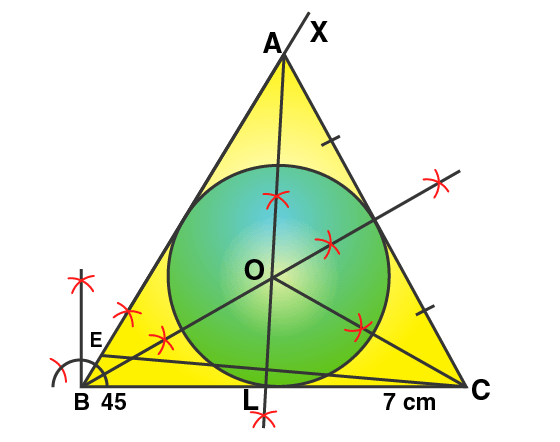Steps of construction:

i) Construction of triangle:

a) Draw a line segment BC = 7 cm

b) At B, draw a ray BX making an angle of 45o and cut off BE = AB – AC = 1 cm

c) Join EC and draw the perpendicular bisector of EC intersecting BX at A.

d) Finally, join AC.

Thus, ΔABC is the required triangle.

ii) Construction of incircle:

e) Draw the angle bisectors of ∠ABC and ∠ACB which intersect each other at O.

f) From O, draw perpendicular OL to BC.

g) With O as centre and OL as radius draw a circle which touches the sides of the ΔABC. This is the required in-circle of ΔABC.

On measuring, we get the radius OL = 1.8 cm

7. Using ruler and compasses only, draw an equilateral triangle of side 5 cm. Draw its inscribed circle. Measure the radius of the circle.

Solution: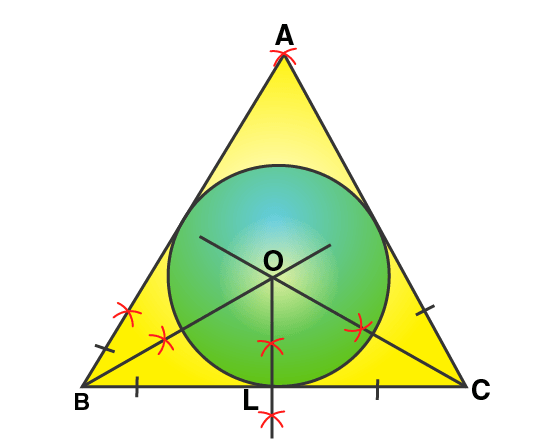Steps of Construction:

i) Draw a line segment BC = 5 cm

ii) With centres as B and C, draw two arcs of radius 5 cm each which intersect each other at A.

iii) Then, join AB and AC.

iv) Draw angle bisectors of ∠B and ∠C which intersect each other at O.

v) Draw OL ⊥ BC, from O.

vi) Now with centre O and radius OL, draw a circle which will touch the sides of ΔABC.

On measuring the radius, we have OL = 1.4 cm.

8. Using ruler and compasses only,

(i) Construct a triangle ABC with the following data:

Base AB = 6 cm, BC = 6.2 cm and ∠CAB = 60o

(ii) In the same diagram, draw a circle which passes through the points A, B and C and mark its centre as O.

(iii) Draw a perpendicular from O to AB which meets AB in D.

(iv) Prove that AD = BD

Solution: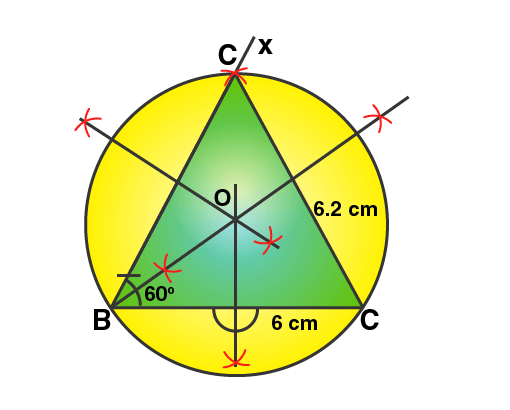Steps of construction:

i) Draw a line segment AB = 6 cm

ii) Draw a ray at A, making an angle of 60o with BC.

iii) With B as centre and radius = 6.2 cm draw an arc which intersects AX ray at C.

iv) Join BC.

Then, ΔABC is the required triangle.

v) Draw the perpendicular bisectors of AB and AC intersecting each other at O.

vi) With centre O, and radius as OA or OB or OC, draw a circle which will pass through A, B and C.

vii) From O, draw OD ⊥ AB.

Proof: In right ΔOAD and ΔOBD

OA = OB [Radii of same circle]

OD = OD [Common]

∆OAD ≅ ∆OBD by RHS congruence criterion.

Hence, by CPCT

9. Using ruler and compasses only construct a triangle ABC in which BC = 4 cm, ∠ACB = 45o and perpendicular from A on BC is 2.5 cm. Draw a circle circumscribing the triangle ABC.

Solution: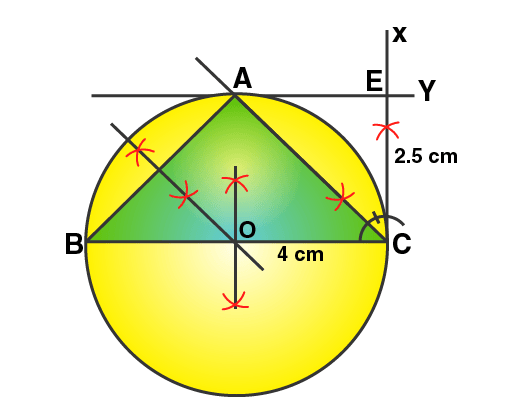Steps of Construction:

i) Draw a line segment BC = 4 cm.

ii) At C, draw a perpendicular line CX and from it, cut off CE = 2.5 cm.

iii) Draw another perpendicular line EY from E.

iv) From C, draw a ray making an angle of 45o with CB, which intersects EY at A.

v) Join AB.

Then, ΔABC is the required triangle.

vi) Draw perpendicular bisectors of sides AB and BC intersecting each other at O.

vii) With centre O, and radius OB, draw a circle which will pass through A, B and C.

On measuring, we have the radius OB = OC = OA = 2 cm.

10. Perpendicular bisectors of the sides AB and AC of a triangle ABC meet at O.

(i) What do you call the point O?

(ii) What is the relation between the distances OA, OB and OC?

(iii) Does the perpendicular bisector of BC pass through O?

Solution: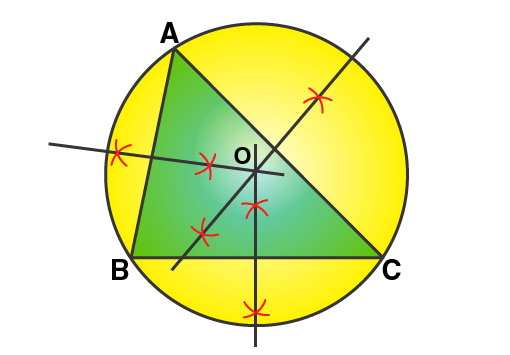i) O is called the circumcentre of circumcircle of ΔABC.

ii) OA, OB and OC are the radii of the circumcircle.

iii) Yes, the perpendicular bisector of BC will pass through O.

11. The bisectors of angles A and B of a scalene triangle ABC meet at O.

i) What is the point O called?

ii) OR and OQ are drawn perpendiculars to AB and CA respectively. What is the relation between OR and OQ?

iii) What is the relation between angle ACO and angle BCO?

Solution: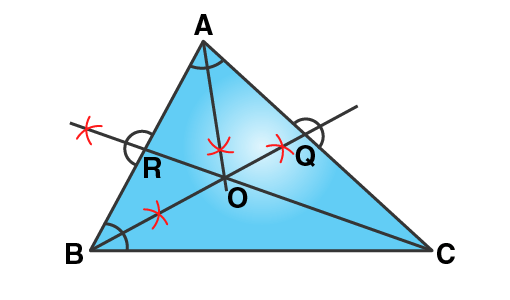i) O is called the incentre of the incircle of ΔABC.

ii) OR and OQ are the radii of the incircle and OR = OQ.

iii) OC is the bisector of angle C

Thus, ∠ACO = ∠BCO

12. i) Using ruler and compasses only, construct a triangle ABC in which AB = 8 cm, BC = 6 cm and CA = 5 cm.

ii) Find its incentre and mark it I.

iii) With I as centre, draw a circle which will cut off 2 cm chords from each side of the triangle.

Solution: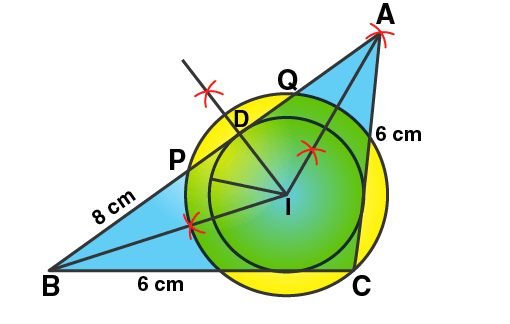Steps of construction:

i) Draw a line segment BC = 6 cm.

ii) Draw an arc with centre B and radius 8 cm.

iii) Draw another arc with centre C and radius 5 cm which intersects the first arc at A.

iv) Join AB and AC.

Thus, ΔABC is the required triangle.

v) Draw the angle bisectors of ∠B and ∠A which intersect each other at I. Then I is the incentre of the triangle ABC.

vi) Through I, draw ID ⊥ AB.

vii) Now from D, cut off DP = DQ = 2/2 = 1 cm

viii) With centre I, and radius IP or IQ, draw a circle which will intersect each side of triangle ABC cutting chords of 2 cm each.

13. Construct an equilateral triangle ABC with side 6 cm. Draw a circle circumscribing the triangle ABC.

Solution:Steps of construction:

i) Draw a line segment BC = 6 cm

ii) Draw two arcs of radius 6 cm with centres B and C, which intersect each other at A.

iii) Then, join AC and AB.

iv) Draw perpendicular bisectors of AC, AB and BC intersecting each other at O.

v) Draw a circle with centre O, and radius OA or OB or OC which will pass through A, B and C.

Then, this is the required circumcircle of triangle ABC.

14. Construct a circle, inscribing an equilateral triangle with side 5.6 cm.

Solution:Steps of Construction:

i) Draw a line segment BC = 5.6 cm

ii) With centres B and C, draw two arcs of 5.6 cm radius each which intersect each other at A.

iii) And, join AB and AC.

iv) Draw angle bisectors of ∠B and ∠C which intersect each other at O.

v) From O, draw OL ⊥ BC.

vi) Now with centre O and radius OL, draw a circle which will touch the sides of ΔABC.

Thus, this is the required circle.

The given solutions are as per the 2019-20 Concise Selina textbook. The Selina Solutions for the academic year 2020-21 will be updated soon.